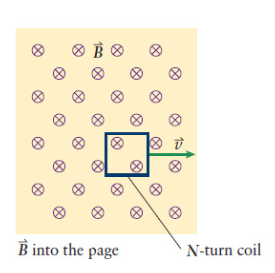# Problem: A square, 60-turn coil 10.0 cm on a side with a resistance of 0.920 Ω is placed between the poles of a large electromagnet. The electromagnet produces a constant, uniform magnetic field of 0.600 T directed into the page. As suggested by the figure below the field drops sharply to zero at the edges of the magnet. The coil moves to the right at a constant velocity of 2.00 cm/s. What is the current through the wire coil before the coil reaches the edge of the field? (Express your answer to two significant figures.)

###### FREE Expert Solution
84% (273 ratings)
###### Problem Details

A square, 60-turn coil 10.0 cm on a side with a resistance of 0.920 Ω is placed between the poles of a large electromagnet. The electromagnet produces a constant, uniform magnetic field of 0.600 T directed into the page. As suggested by the figure below the field drops sharply to zero at the edges of the magnet. The coil moves to the right at a constant velocity of 2.00 cm/s. What is the current through the wire coil before the coil reaches the edge of the field? (Express your answer to two significant figures.)